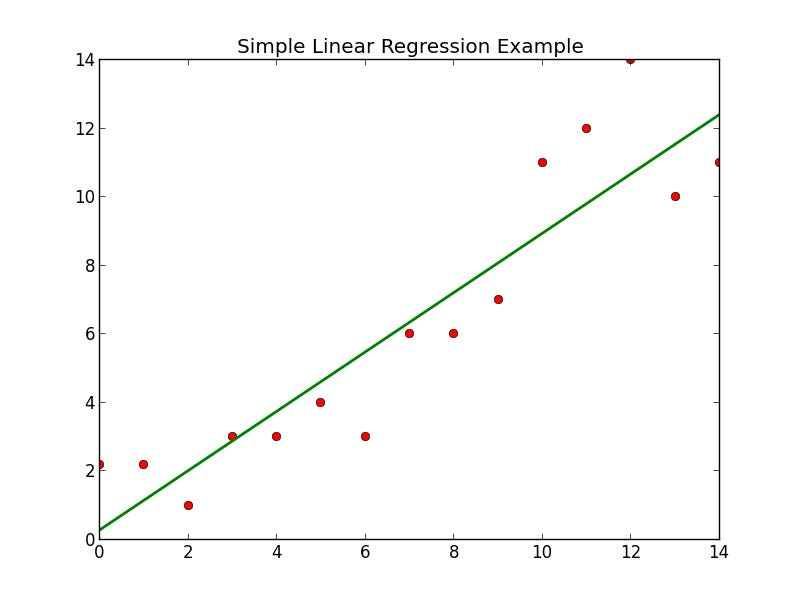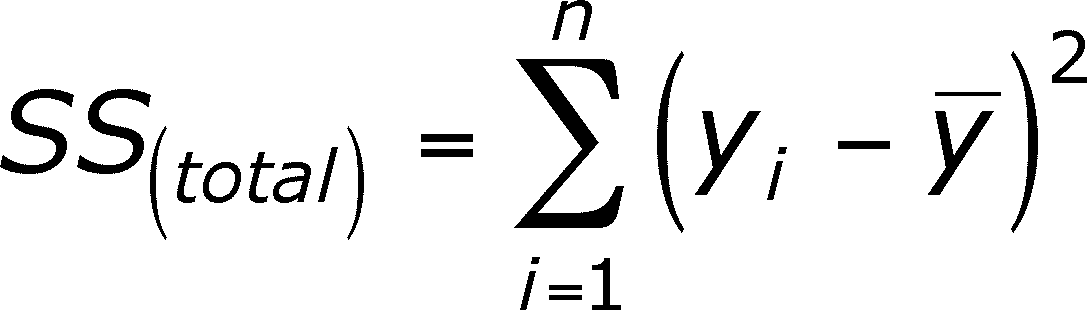# Machine Learning Model: Simple Linear Regression

One of the ways to accurately describe Machine Learning is it’s the domain of figuring out mathematical optimization for real-world problems. Yes, it’s all maths down the road! You pick a problem that you deeply care about solving, you find suitable data that you think will help you get insights on that problem, you pick the most favorable model for the situation and then hop on to the later stages. In this article, I will focus on one particular machine learning model, which also happens to be the simplest out there, cover the theory and then show how to apply it.

Sometimes when you are trying to solve a real world problem using machine learning, you may want to examine whether if certain factors have any correlation with a certain impact. For instance, you may want to examine whether an increase in consumption of junk food has any correlation with the number of people diagnosed with cholesterol in a certain place over a certain period of time. In the particular example, the number of people consuming junk food would be a predictor variable (independent variable x), which is free to change, and the number of people being diagnosed with the disease would be the response variable (dependent variable y) responding to the change in x according to our assumption.

Here I must point out that correlation does not necessarily mean causation.
“The assumption that A causes B simply because A correlates with B is often not accepted as a legitimate form of argument”. A classic correlation vs causation example is that smoking may be correlated with alcoholism, but doesn’t cause alcoholism. Keep that in mind.

#### Moving on, what is a Simple Linear Regression?

A linear regression is the approach of forming a relationship between the independent and dependent variables. The simplest situation you can encounter is when you are to examine a single predictor variable (independent variable x) for a certain response. In other words, you would want to check whether if a single action has any relationship to a certain response. This is called a simple linear regression.

The process is pretty straightforward. We will simply try to come up with a straight line that will best fit our data. At this point, you may feel like brushing up your high school maths. Don’t worry, I have got you covered.(On the left) Equation of a straight line. y is the dependent variable and x is the independent variable. The gradient is the slope of the line which determines how steep or flat the line is and the y-intercept is basically where the line cuts the y-axis. (On the right) A line that best fits the data.

Before we begin writing our algorithm, it is necessary for us to understand how is our algorithm able to figure out the equation for the straight line that will be the best fit just by looking into our data.

If you look at the diagram on the left, you can see something called the vertical offset, which is the difference between the actual data and the predicted straight line model.

Our algorithm takes the squared sum of all these vertical offsets, i.e differences, and comes up with the line for which this sum is the minimum. This is the crux of our algorithm.The best fit line is the one for which the SS value will be the minimum.

In my previous article, I discussed the systematic steps of preprocessing the data for machine learning. I discussed why it is necessary and how you can split your data set into training set and test set. The data preprocessing template is available here for use.

Once you have the data ready, we will perform linear regression on our data set. We don’t have to perform the operation manually. The tedious task is made easy by a library called sklearn.linear_model, which has a class perfect for the job called LinearRegression. Obviously!

`from sklearn.linear_model import LinearRegression`

If you remember from my previous article, the next step naturally is to create an object of that class to call the functions of that class. We will name our object regressor.

`regressor = LinearRegression()`

Now our regressor needs to train on our dataset. The training is also called fit. We will feed our two training sets, X_train and y_train, as its parameter. (Please kindly refer to my previous article if this confuses you)

`regressor.fit(X_train, y_train)`

We are basically telling the machine to use the linear regression model and learn from our set of data points in our training sets. The machine is learning!

Now that our regressor object has learned from our training sets, we would want to examine how accurately it can predict new observations. Very simply, we will use a method called predict, available in the LinearRegression class. As its parameter, we will feed the X_test so as to see how well it can predict the response for them (corresponding dependent variable Y). For now, let’s just call them y_pred.

`y_pred = regressor.predict(X_test)`

This will give us a series of predicted data. Here, y_pred are the predicted values, while our y_test data are the actual values. We can compare both sets to evaluate how well or worse our model has performed.

We can then go on to transform our results into visual graphs. We will basically scatter plot our data and plot the best fit line. For this task, we will use the matplotlib library which is one of the most popular Python 2D plotting library.

`import matplotlib.pyplot as plt`

We want a scatter plot, so will invoke the scatter method. Initially, we want to scatter plot our training sets — X_train and y_train. We may decide to represent them by the color blue.

`plt.scatter(X_train, y_train, color = 'blue')`

In the midst of our scattered data, we want to plot, not scatter plot, our best fit line. On the same graph, the coordinates of the best fit line are the x-coordinates (X_train) and the corresponding predicted values. We had previously predicted the values for X_test. Simply by replacing X_test with X_train, we can find corresponding predicted values for it in similar manner. The code that follows is pretty self-explanatory:

`plt.plot(X_train, regressor.predict(X_train), color = 'red')`

We are basically telling to plot a line with X_train as x-coordinates and corresponding predicted values for each of the data points in X_train as y-coordinates. Let the best fit line be in red.

Now to just display the figure, we will use:

`plt.show()`

In the diagram above, the blue dots are the real values, while the data points in red are the predicted values. Blue dots above the red best fit line tells us that the actual value is higher than our predicted value, those lower tells us that the actual value is lower than our predicted value, and those that coincides with our best fit line have been accurately predicted by our model. It is based on these training set that our model (the best fit line) has trained. To figure out whether our model has fared well or not, we will draw another graph, this time we will keep the best fit line as it is, but scatter plot our test data (X_test) instead.

`plt.scatter(X_test, y_test, color = 'blue')plt.plot(X_train, regressor.predict(X_train), color = 'red')plt.show()`

If more of the plots coincide with our best fit line or is considerably closer to it, it is fair to say that our model has performed well.

And just by that you will have created your own machine learning model. You have made your model train on the given dataset and find correlation between the independent and dependent variables, represented by the best fit line. The best fit line can then be used to make future predictions.

Try applying this model to these following situations:

1. High school GPA vs College entrance test score
2. Lean body mass vs Muscle strength
3. Money spent on advertising vs Total Sales
4. Years of experience vs Salary
5. Physical exercise (in mins) vs Cholesterol level

Goodluck!